# Pressure in liquids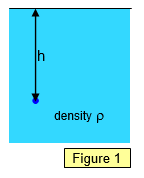The pressure in a liquid depends on three things:
(a) the depth within the liquid (measured vertically from its surface)
(b) the density of the liquid (assumed uniform)
(c) the intensity of the gravitational field at that point (g)

Pressure at a depth h in a liquid of density r = hrg

Notice that the total pressure at a depth h will be that due to the liquid (hrg) PLUS that due to the atmosphere above it.

Example problems
The pressure of the atmosphere is about 105 Pa. Calculate the depth below the surface of water where the total pressure (atmospheric + water pressure) is twice that amount i.e 2x105 Pa. (Density of water = 1000 kgm-3)

Exces pressure due to water = 105 Pa = hrg = h x 1000x9.8
Therefore h = 102/9.8 = 10.2 m

## Two important properties of the pressure in a liquid:

(a) Pressure at the same level in a liquid is the same
The pressure at any two points at the same level in a liquid is the same since the depth below the surface is the same.
(b) Pressure at a point is the same in all directions
Since the pressure at all points at the same depth is the same it follows that that the pressure at a point is the same in all directions.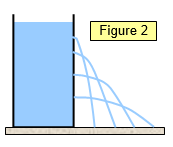### Can with holes

A very simple demonstration of the increase in pressure in a liquid with depth is to use a can with a series of vertical holes drilled in the side. If the can is filled with water the water will stream out of the holes with that emerging from the lowest hole spurting out most rapidly and travelling the furthest because the pressure there is greatest.
(See Figure 2)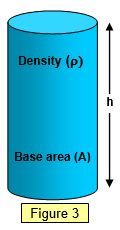### Derivation of liquid pressure formula from the weight of a column of liquid

Pressure at the base is the weight of the liquid column divided by the cross sectional area.

Therefore pressure = Vrg/A = hArg/A = hrg (See Figure 3)

### Problems of divers and the bends

When diving the dissolved gases in the blood are compressed. As you rise to the surface again these gases expand due to the reduced pressure. Rapid rise causes rapid expansion and resulting pain known as the bends. Decompression is needed. This is the slow reduction of the pressure on the body to release dissolved gases from the blood slowly. A world famous diving location is the 'President Coolidge' an American troop ship sunk off the coast of Santo in Vanuatu. Decompression takes over an hour for divers rising from the stern of the ship.

### Height of the atmosphere assuming uniform density

We can find the height of the atmosphere by assuming it was of uniform density equal to the density of air at sea level. We take the height of the column of mercury in a mercury barometer at sea level to be 0.76 cm of mercury.

Density of mercury = 13600 kgm-3, density of air at sea level = 1.2 kgm-3
Therefore height of the atmosphere = [13600/1.2] x 0.76 = 8220 = 8.2 km.

### Cave system and angled tubes – it is the vertical height that matters

The pressure in a liquid depends on the vertical height below its surface and so the pressure at the bottom of all the tubes shown in Figure 4 is the same.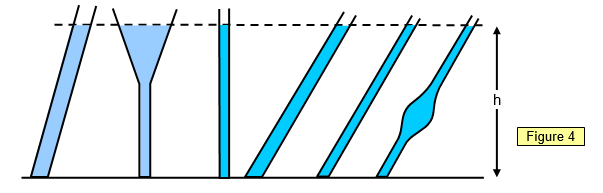From this it follows that the pressure at points of equal distance below the ground in a complex water filled cave system will all be the same. (See Figure 5)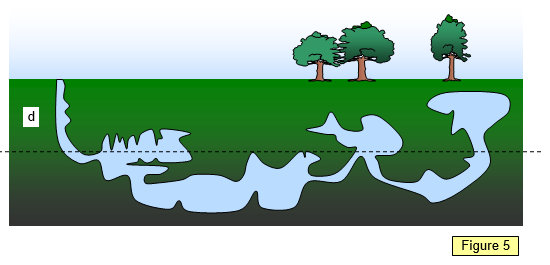### Different shaped water towers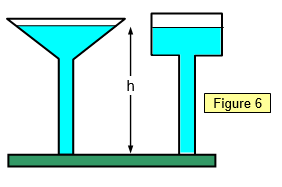The pressure at the base of each water tower is the same since it only depends on the vertical height difference between the base and the water surface. What is different is the rate at which the pressure falls a when a certain volume of liquid flows out of each tower. The water level in the tower on the left will drop by a smaller amount that that in the tower on the right meaning a smaller change in pressure at the base.

### Chocolate blancmange in a barrel - an analogy with the mercury barometer

Imagine a barrel full of chocolate blancmange with a wooden lid that just fits inside the top of the barrel and rests on the surface of the blancmange. This lid has a hole in its centre to which is fitted a vertical glass tube. If you now press down on the lid the blancmange is forced up the tube. The harder you press the further up the tube it goes. This is just like the air pressing down on the mercury in the open dish of the simple barometer.

### Strength and height of a granite column

The pressure exerted by the weight of the rock will determine how tall a rock column can be before it shatters under its own weight. This will occur at the base of the column where the pressure is greatest. This also happens with brick structures such as the tower in St Mark's square in Venice, which collapsed due to the bricks at the base failing.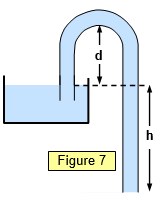### The siphon

Water can be made to flow uphill through a tube and over a barrier if the lower open end of the tube is below the water level of the water in the container and the tube is completely full of water. The pressure in the water at the open end of the base of the siphon is atmospheric + hrg. This is greater than the air pressure at that point and so water flows out. (See Figure 7).

Clearly water will not flow if the height of the top of the tube above the water surface (d) is greater than the atmospheric pressure. The presence of bubbles of air in the siphon pipe cause problems with the operation of a siphon.

### Pressure on sea bed with a boat passing overhead

As long as the depth of the water does not change the pressure below a boat will be exactly the same as if the boat was not there.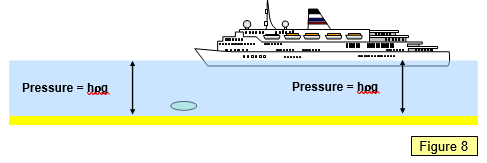A VERSION IN WORD IS AVAILABLE ON THE SCHOOLPHYSICS USB